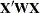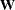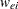ID Statement
ID variables ;

The ID statement specifies which quantities to include in the OUT= data set from the OUTPUT statement in addition to any statistics requested in the OUTPUT statement. If no ID statement is given, the GLIMMIX procedure includes all variables from the input data set in the OUT= data set. Otherwise, only the variables listed in the ID statement are included. Automatic variables such as _LINP_, _MU_, _VARIANCE_, etc. are not transferred to the OUT= data set unless they are listed in the ID statement.

The ID statement can be used to transfer computed quantities that depend on the model to an output data set. In the following example, two sets of Hessian weights are computed in a gamma regression with a noncanonical link. The covariance matrix for the fixed effects can be constructed as the inverse of.is a diagonal matrix of theor, depending on whether the expected or observed Hessian matrix is desired, respectively.

proc glimmix;
class group age;
model cost = group age / s error=gamma link=pow(0.5);
output out=gmxout pred=pred;
id _variance_ wei woi;
vpmu  = 2*_mu_;
if (_mu_ > 1.0e-8) then do;
gpmu  =  0.5  * (_mu_**(-0.5));
gppmu = -0.25 * (_mu_**(-1.5));
wei   = 1/(_phi_*_variance_*gpmu*gpmu);
woi   = wei + (cost-_mu_) *
(_variance_*gppmu + vpmu*gpmu) /
(_variance_*_variance_*gpmu*gpmu*gpmu*_phi_);
end;
run;

The variables _VARIANCE_ and _MU_ and other symbols are predefined by PROC GLIMMIX and can be used in programming statements. For rules and restrictions, see the section Programming Statements.